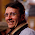## Wednesday, December 30, 2009

### Selecting the median in an array

Ok, this is a typical "easy" interview question: you are given an array of integers, select the median value. What is the optimal solution?

1.Various solutions are:

1) Sort ant take the element in the middle; O(n log n) and it takes additional space O(n)

2) QuickSelect which you find in any Algo book is linear in average, but still takes O(n) space

3) A linear solution given by Cormern and other, which I cited in a previous post. It still takes additional O(n) space.

What if I want to have this in place?

2.It can be done in O(n log n) time and O(1) additional space using binary search.

Assume that the median is contained in the range [l,r]. With a single scan of the array we can sample a random value among the elements of the array contained in [l,r]. Then we just count the number of values less than x in order to decide if the median is in [l,x] or [x,r]. This solution does not modify the array.

3.that's classical quickselect. Any linear time solution ?

4.Hum, probably I misunderstood the question... is the algorithm allowed to modify the input array?
If the answer is no then achieving O(n) time in the RAM model is probably an open problem and is actually impossible in the comparison-based model (see T.Chan SODA09 on that problem). If the answer is yes, the classic selection algorithm inspired by quicksort is O(n) expected time and is already inplace.

5.No it is not allowed

6.In practice, if the integers are 32-bit words, i would use a radix-based approach. With a single scan of the array build a table with 256 entries, such that T[i] stores the number of elements whose topmost 8 bits are i. Then you can trivially locate the bucket containing the median, which gives its first 8 bits. Reiterating the same scheme compute the median with 4 scan of the array and 256 words of extra space. Clearly, the size of the table need to be tuned to make the algorithm as fast as possible in practice.